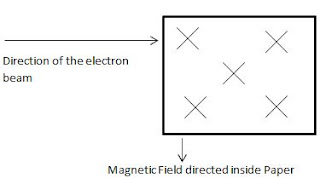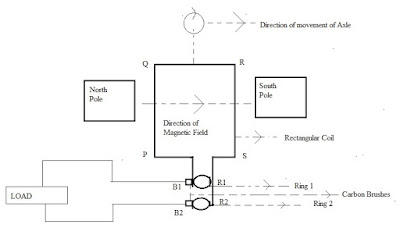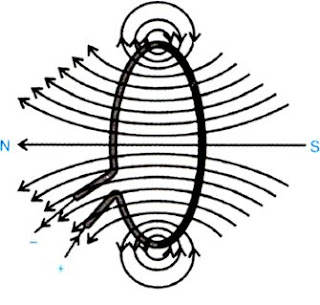#### Extra Questions for Class 10th: Ch 13 Magnetic Effects of Electric Current (Science) Important Questions Answer Included

Very Short Answer Questions (VSAQs): 1 Mark

Q1. Name the measure used in domestic circuiting to prevent shock from leakage of current.
Earthing

Q2. An alternating current has frequency of 50Hz. How many times it has changed its direction in one second?
100 times, 50Hz frequency means AC generator completes 50 cycles in 1 second.

Q3. Name the rule which is used to find the direction of current carrying conductor in a wire.
Right hand thumb rule or Maxwell’s thumb rule

Q4. State the value of potential difference between the live wire and the neutral wire in our country.
220V

Q5. Mention the angle between a current carrying conductor and magnetic field for which the force experienced by this current carrying conductor placed in magnetic field is largest.
90°

Short Answer Questions-I (SAQs-I): 2 Marks

Q1. PQ is a current carrying conductor in the plane of the paper as shown in the fig. Mention the direction of magnetic fields produced by it at points A and B. Given : r1 < r2 where will the strength of the magnetic field be larger ? Justify your answer in each case.Strength of magnetic field will be larger at A as the strength of magnetic field decreases when the distance is increased. Direction of magnetic field lines at A as well as B is anticlockwise because field lines are concentric circles and direction is determined by Right hand thumb rule.

Q2. How the strength of the magnetic field due to current carrying conductor does depends upon?

(i) distance  of the test point from the conductor
(ii) Magnitude of the Current flowing through the conductor

Q3. A current carrying wire entering a magnetic field experiences a force. In the diagram given below, a beam of electron is entering a magnetic field experience a force. From the below diagram, describe the direction of the magnetic field and direction of the current.The direction of current is taken opposite to the direction  of the electron beam.  Now, current direction is from right to left.  The direction of magnetic field is given inside the paper. Now, if we apply Fleming’s left hand rule, we can obtain the direction of electron which is shown in the figure drawn.

Q4. Write two reasons why appliances are connected in parallel in house hold circuiting?

(i) Each appliances are at same potential
(ii) Each appliances can get individual switch

Q5.  Can two magnetic lines of force intersect each other ? Give reasons in support of your answer.

No, two magnetic lines of force never intersect each other. If two magnetic lines of force intersect each other than at the point of intersection there will be two different directions of magnetic field which is not possible.

Short Answer Questions-I (SAQs-II): 3 Marks

Q1. What is an electromagnet ? How can we determine north and south pole of an electromagnet with the help of magnetised iron bar.

An electromagnet is a device which generates magnetic field when electric current passes through it.
It works on the principle of magnetic effect of current.
To suspend magnetised bar and identify its north and south poles.
To find the polarity of electromagnet using the property-like poles repel. A bar magnet whose north and south pole is known, is brought near the poles of electromagnet. If north pole of bar magnet is deflected, that is south pole of electromagnet.

Q2. State and explain Fleming's right hand rule for the direction of induced current.

The direction of induced current in a straight conductor is given by Fleming's right hand rule. It states that , when we spread the thumb, forefinger and the middle finger of our right hand at right angle to one another  if the forefinger points in the direction of magnetic field, thumb gives the direction of force, then the direction in which the middle finger points, gives the direction of induced current.

Q3. Explain briefly two different ways to induce current in a coil. State the rule which determines the direction of induced current.

Two ways to induce current in a coil : (i) If a coil is moved in a magnetic field.
By bringing a bar magnet close to the coil or taking away from it.
The direction is obtained by Flemings right hand rule.

Q4. Write one difference between direct current and alternating current. Which one of the two is mostly produced at power stations in our country ? Name one device which provides alternating current. State one important advantage of using alternating current.

The polarity of current remains same and current flows in same direction.  The direction of Alternating current changes after a regular time interval.
Most power stations produce AC in India/an AC generator.
By using Alternating current, the power can be transmitted easily using transformers.

Long Answer Questions (LAQs): 5 Marks

Q1. State the principle of an electric generator. Draw a well labeled diagram of AC generator. Describe difference between AC and DC generator is design.

Electric generator is based on the principle of electromagnetic induction.The brushes are attached permanently to the rings in AC generator. In DC generator, the split rings are present which changes carbon brushes at each half rotation.

Q2. What are magnetic field lines ? List any two characteristics of field lines. Draw the pattern of magnetic field lines due to a current carrying circular loop